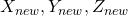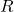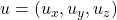# Transform Axes Jun 2021

Generally transforming coordinate axes

Here is yet another presentation about a VBA function that was recoded in Python.

In structural engineering, we must constantly deal with axes transformations.  Mostly we have right handed coordinates (RHC), but sometimes left handed coordinates (LHC). For example, LHCs might occur when South or East map coordinates are used. Certain engineering software packages use LHC.

A few quick questions:

• How many XYZ orientations are there?
(48)
• How many RHC XYZ orientations are there?
(24)
• How many LHC XYZ orientations are there?
(24)

Conceptual proof:

• Label the directionsas 1, 2, 3
• Label the directionsas 4, 5, 6• Now imagine transformingto.
• Let’s say(∴ 1) and(∴ 3)
• We could use the two digit notation  for the new system orientation
• There are 4 orientations starting with 1:
• :• :• :• :• : isn’t legal.andmust have different directions
• : isn’t legal.andcannot be in opposite directions
• Consider then two digit orientations 2_, 3_, 4_, 5_, 6_
• For each of these we can have four orientations
• Therefore, there are 24 RHC orientations; 6 choices fortimes 4 choices for• Therefore, there are 24 RHC orientations
• Then allow RHC or LHC orientations
• Therefore, there are 24 LHC orientations
• Therefore, there are 48 total orientations

This makes it almost trivial to think through the transformation desired.

Given a point, to make the RHC transformation we simply execute a single rotation about a single vector:

• to produce : rotatezero degrees about vector## no change
• to produce : rotate180 degrees about vector• to produce : rotate90 degrees about vector• to produce : rotate270 degrees about vector• to produce : rotate180 degrees about vector• to produce : rotate180 degrees about vectorTo make the LHC transformation we negate the Z-axis coordinate of the output point after rotating the point about the vector.The Final Function (with PDF)
For my transformation function, I predetermined the vectors and rotations for the 24 RHC orientations.

Using PyXLL, the function is callable from Excel.

##### Another example
Rotation matrix and rotation equation for :(∴ 2) and(∴ 3)In row 19, notice that a single PyXLL function call takes a single orientation input , calculates the rotation matrix once, and performs a block of point transformations in a single matrix equation. Yep, that’s a screenshot from an iPhone.The matrix of a proper rotationby anglearound the axis, a unit vector with, is given by:whereis the Levi-Civita symbol with